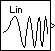# Chirp

Generate swept-frequency cosine (chirp) signal

• Library:
• DSP System Toolbox / Sources

•## Description

The Chirp block outputs a swept-frequency cosine (chirp) signal with unity amplitude and continuous phase. To specify the desired output chirp signal, you must define its instantaneous frequency function, also known as the output frequency sweep. The frequency sweep can be linear, quadratic, or logarithmic, and repeats once every Sweep time by default. For a description of the algorithms used by the Chirp block, see Algorithms.

## Ports

### Output

expand all

Swept-frequency cosine (chirp) signal. In `Linear`, `Logarithmic`, and `Quadratic` modes (set by the Frequency sweep parameter), the block outputs a swept-frequency cosine with instantaneous frequency values specified by the frequency and time parameters. In ```Swept cosine``` mode, the block outputs a swept-frequency cosine with a linear instantaneous output frequency that may differ from the one specified by the frequency and time parameters.

Data Types: `single` | `double`

## Parameters

expand all

The type of output instantaneous frequency sweep, fi(t): `Linear`, `Logarithmic`, `Quadratic`, or ```Swept cosine```. For more information, see Shaping the Frequency Sweep and Algorithms.

#### Limitations

When you want a linearly swept chirp signal, we recommend that you use a `Linear` frequency sweep. Though a `Swept cosine` frequency sweep also yields a linearly swept chirp signal, the output might have unexpected frequency content.

• The swept cosine sweep value at the Target time is not necessarily the Target frequency. This is because the user-specified sweep is not the actual frequency sweep of the swept cosine output, as noted in Output Computation Method for Swept Cosine Frequency Sweep. See the table Instantaneous Frequency Sweep Values for the actual value of the swept cosine sweep at the Target time.

• In Swept cosine mode, do not set the parameters so that 1/Tsw is much greater than the values of the Initial frequency and Target frequency parameters. In such cases, the actual frequency content of the swept cosine sweep might be closer to 1/Tsw, far exceeding the Initial frequency and Target frequency parameter values.

The Sweep mode parameter determines whether your sweep is unidirectional or bidirectional, which affects the shape of your output frequency sweep (see Shaping the Frequency Sweep). The following table describes the characteristics of unidirectional and bidirectional sweeps.

Sweep Mode Parameter SettingsSweep Characteristics

`Unidirectional`

• Lasts for one Sweep time, Tsw

• Repeats once every Tsw

`Bidirectional`

• Lasts for twice the Sweep time, 2Tsw

• Repeats once every 2Tsw

• First half is identical to its unidirectional counterpart.

• Second half is a mirror image of the first half.

The following diagram illustrates a linear sweep in both sweep modes. For information on setting the frequency values in your sweep, see Setting Instantaneous Frequency Sweep Values.For `Linear`, `Quadratic`, and ```Swept cosine``` sweeps, the initial frequency, f0, of the output chirp signal. You can specify the Initial frequency (Hz) as a scalar, greater than or equal to zero. For `Logarithmic` sweeps, Initial frequency is one less than the actual initial frequency of the sweep. Also, when the sweep is `Logarithmic`, you must set the Initial frequency to be less than the Target frequency.

Tunable: Yes

For `Linear`, `Quadratic`, and `Logarithmic` sweeps, the instantaneous frequency, fi(tg), of the output at the Target time, tg. You can specify the Target frequency (Hz) as a scalar, greater than or equal to zero. For a `Swept cosine` sweep, Target frequency is the instantaneous frequency of the output at half the Target time, tg/2. When Frequency sweep is `Logarithmic`, you must set the Target frequency to be greater than the Initial frequency.

Tunable: Yes

For `Linear`, `Quadratic`, and `Logarithmic` sweeps, the time, tg, at which the sweep reaches the Target frequency, fi(tg). For a `Swept cosine` sweep, Target time is the time at which the sweep reaches 2fi(tg) - f0. Target time must be a scalar that is greater than or equal to zero, and less than or equal to Sweep time , ${T}_{sw}\ge {t}_{g}$.

Tunable: Yes

In `Unidirectional` Sweep mode, the Sweep time, Tsw, is the period of the output frequency sweep. In `Bidirectional` Sweep mode, the Sweep time is half the period of the output frequency sweep. Sweep time must be a scalar that is greater than or equal to Target time, ${T}_{sw}\ge {t}_{g}$.

Tunable: Yes

The phase, ${\varphi }_{0}$, of the cosine output at t=0; ${y}_{chirp}\left(t\right)=\mathrm{cos}\left({\varphi }_{0}\right)$. You can specify the Initial phase (rad) as a scalar that is greater than or equal to zero.

Tunable: Yes

The sample period, Ts, of the output. The output frame period is MoTs, where Mo is the number of samples per frame.

The number of samples, Mo, to buffer into each output frame, specified as a positive integer scalar.

The data type of the output, specified as single precision or double precision.

## Block Characteristics

 Data Types `double` | `single` Multidimensional Signals `No` Variable-Size Signals `No`

expand all

## Algorithms

expand all

The Chirp block uses one of two formulas to compute the block output, depending on the Frequency Sweep parameter setting. For details, see the following sections.

Watch now